# What is the Final Charge on the Capacitor?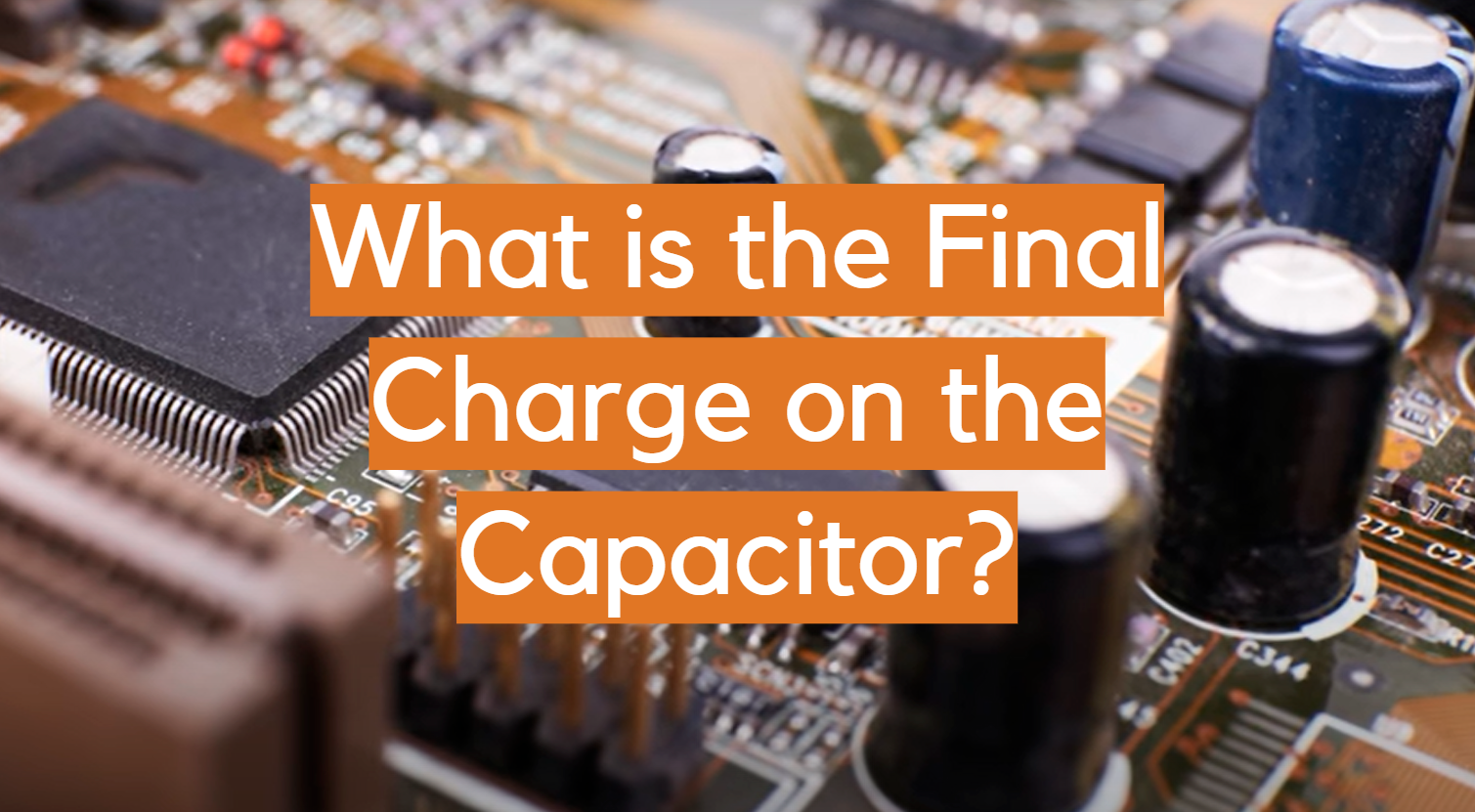When you are looking to buy a capacitor, one of the most important factors that you need to consider is the final charge. This is the amount of energy that the capacitor can store before it needs to be recharged.

If you are looking for a capacitor that can store a lot of energy, then you need to look for one with a high final charge.

Many factors affect the final charge of a capacitor, so it is important to understand all of them before making your purchase.

For instance, one of the most important factors that affect the final charge on a capacitor is its size. The larger the capacitor, the more energy it can store. This is why capacitors come in different sizes; so that they can be used for different purposes. If you are looking for a capacitor to use in an electronic device, then you need to make sure that it is large enough to store all of the energy that the device will use.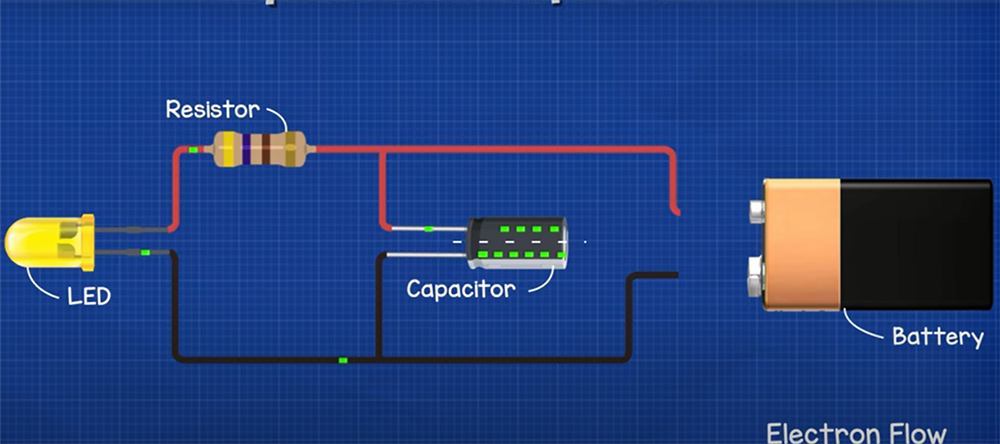In this blog post, we will discuss what the final charge is and how it affects your purchase decision. We will also provide some tips on how to choose the right capacitor for your needs!

## How Do You Calculate The Final Charge Of A Capacitor?

You can calculate the final charge of a capacitor using the equation: Q = C * V

Where Q is the charge in coulombs, C is the capacitance in farads, and V is the voltage in volts .

So, if you have a capacitor with a capacitance of 100 microfarads (0.0001 Farads) and you apply a voltage of 12 volts to it, the final charge on the capacitor will be:

Q = 0.0001 * 12 = 0.0012 Coulombs or 1200 Microcoulombs (1200 uC)

To calculate the charge on a capacitor, you need to know two things: the capacitance of the capacitor and the voltage applied to the capacitor.

The capacitance is a measure of how much charge a capacitor can store and is measured in Farads. The voltage applied to the capacitor determines how much charge is actually stored on the capacitor plates.

You can think of a capacitor as a bucket that can hold a certain amount of water (the capacitance). The more water you put in the bucket (the higher the voltage), the more water it will hold. If you have a very large bucket (high capacitance), it will take more water (higher voltage) to fill it up.

The final charge on the capacitor is directly proportional to both the capacitance and the voltage applied to the capacitor. So, if you double either one, you will double the final charge. If you halve either one, you will halve the final charge.

This is a very important concept in electronics because it allows us to control the amount of charge on a capacitor by simply controlling the voltage applied to it. This is how we can use capacitors to store energy in electric circuits.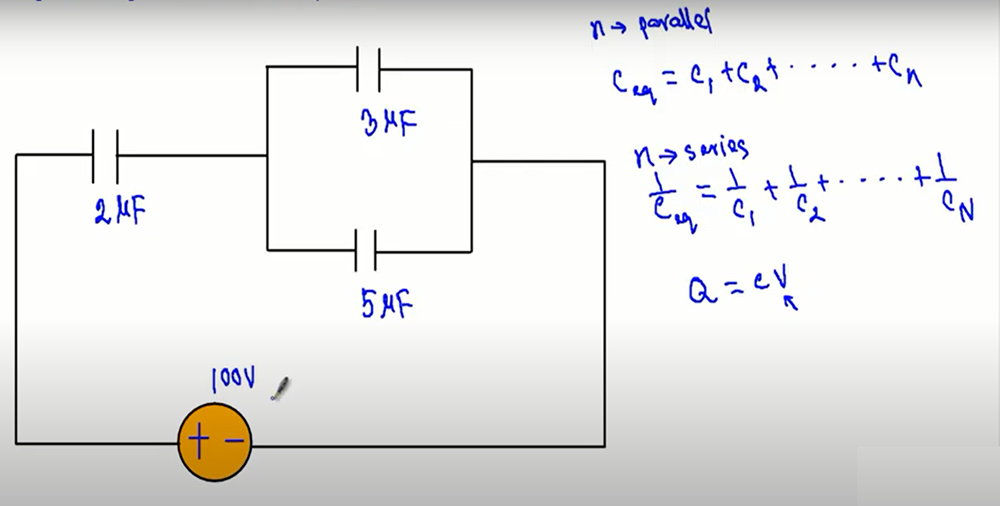By applying a voltage across the plates of a capacitor, experts can create an electric field that stores energy in the form of electrostatic potential energy. When we discharge the capacitor by allowing current to flow through it, that stored energy is converted into kinetic energy in the form of electricity.

## What Is The Charge On The Capacitor When It Is Fully Charged?

When the current through a capacitor is zero, it indicates that it is fully charged.

The phrase “fully charged” refers to a condition in which the voltage of the capacitor matches the battery’s voltage .

This occurs because the capacitor has reached an equilibrium state in which the amount of charge flowing into it is equal to the amount of charge flowing out.

At this point, the capacitor is said to be “fully charged” and its voltage will be equal to the applied voltage.

## What Is Final Capacitor Voltage?

The final capacitor voltage is the voltage that is applied to the capacitor when it is fully charged. This voltage is determined by the battery voltage and the resistor values in the circuit .

The formula for calculating the final capacitor voltage is: Vc = Vb * (Rt / (Rt + Re))

Where Vc is the final capacitor voltage, Vb is the battery voltage, Rt is the total resistance of the circuit, and Re is the effective resistance of the capacitor.

For example, if you have a 12-volt battery and a 100kohm resistor in series with a 100nF capacitor, then your final capacitor voltage would be:

Vc = 12 * (100000 / (100000 + 100)) = 11.99 volts

As you can see, the final capacitor voltage is very close to the battery voltage.

This is because the 100nF capacitor has a very high effective resistance (Re) and therefore does not allow much current to flow through it.

The final capacitor voltage will be lower if the circuit has lower total resistance (Rt) or a higher capacitance (C).

## How Do You Find The Charge On A Capacitor?

To find the charge on a capacitor, you need to know two things: the capacitance of the capacitor and the voltage across it. The capacitance is a measure of how much charge the capacitor can store. The voltage is a measure of the potential difference between the plates of the capacitor.

## What Is The Charging Current?

The current that enters a capacitor after a voltage is first applied . The current is equal to the capacitance times the applied voltage divided by the time constant of the circuit. The current decreases as the capacitor charges and eventually becomes zero.

The time constant is a measure of how long it takes for the capacitor to charge or discharge. It is equal to the product of the capacitance and resistance.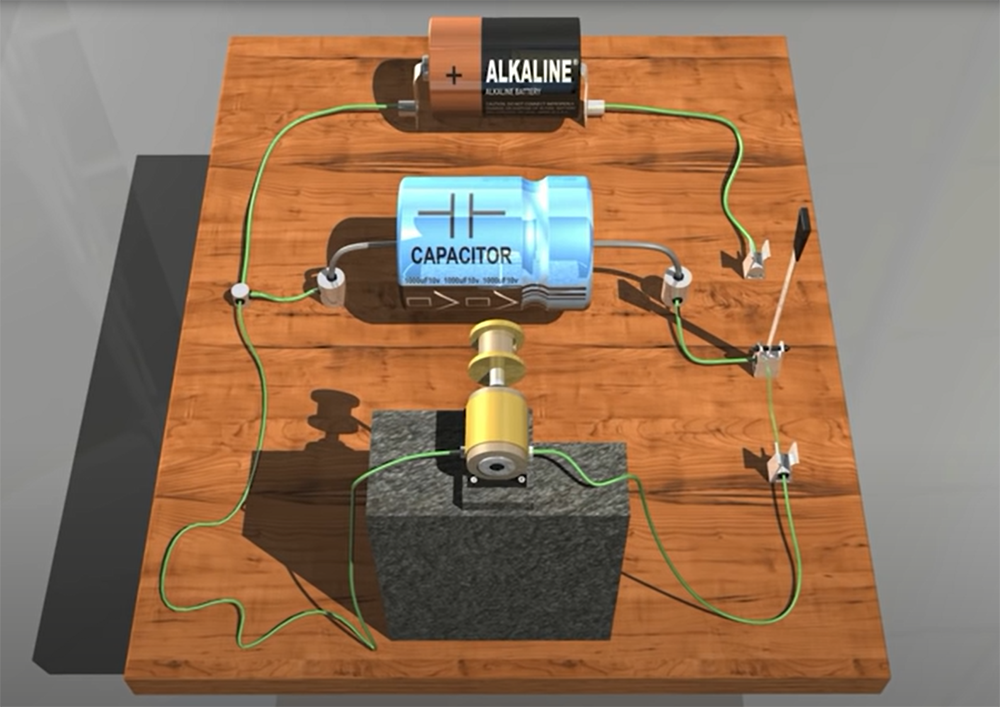In a circuit with an inductor and a capacitor, the time constant is equal to the inductance divided by the capacitance.

When you first apply a voltage to a capacitor, there is a large charging current. This charging current decreases as the capacitor charges and eventually becomes zero.

## How Do You Find The Charge On A Capacitor In Series?

Pairs of identical capacitors connected in series always have an equal amount of charge on their plates.

The voltage drop across the capacitor is determined by the value of the capacitor alone as V = Q ÷ C since the charge (Q) is constant and equal.

## How Do You Charge A Capacitor?

Charging a capacitor is done by connecting it to a battery or power supply so that the electrons can flow from one plate to the other. The amount of charge that flows into the capacitor depends on the voltage of the battery and the capacitance of the capacitor.

It’s straightforward to charge a capacitor. A capacitor is charged by being connected to a DC voltage supply. This may be a battery or a DC power source.

When the capacitor is linked to a DC voltage supply, it will charge up to the level of electricity that the DC voltage source is providing. So, if a 9-volt battery is used with a capacitor, it will quickly charge to 9 volts.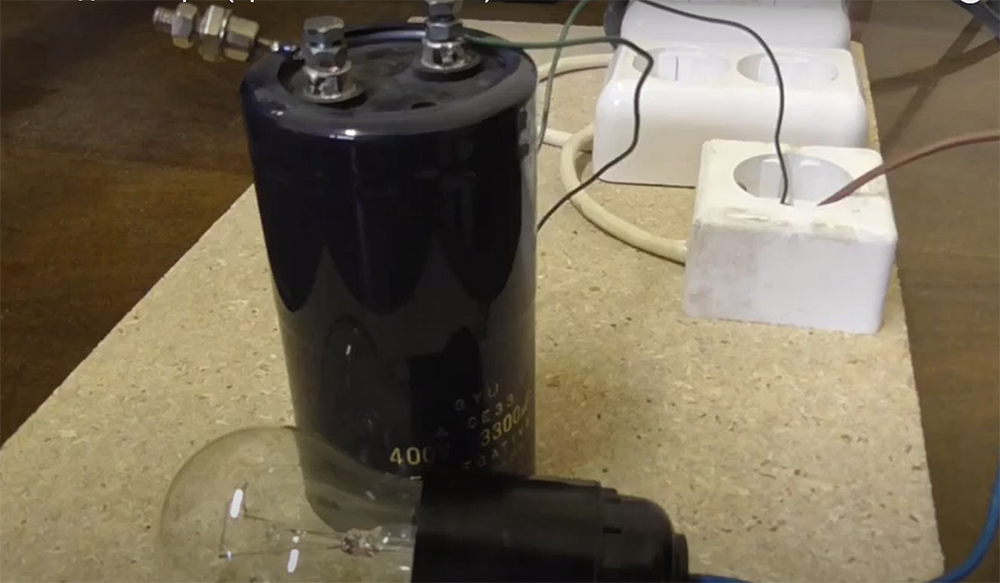A capacitor connected to a DC power supply outputting 15 volts will charge up to 15 volts. All that has to be done is to connect the positive side of the DC voltage source to the positive side of the capacitor, connections are made, and the capacitor will start to fill with electrons.

The time it takes to charge a capacitor depends on two factors:

• The voltage of the DC power source;
• The capacitance of the capacitor;

With a higher voltage, the capacitor will charge more quickly.

With a lower capacitance, it will take longer to fill the plates with electrons and achieve a full charge .

## What Is Charging And Discharging Of Capacitors?

Charging and discharging of capacitors is the process of transferring energy between a capacitor’s plates through an external circuit. This can be done with either a DC or AC power source.

Discharging is when the capacitor releases its stored energy back into the circuit. This happens when the capacitor is disconnected from the power source and there is no longer a flow of electrons to maintain the charge on the plates.

The time it takes for a capacitor to discharge depends on three factors:

• The size of the resistor;
• The voltage of the battery;
• The capacitance of the capacitor;

A higher value resistor will cause the discharge to happen more slowly because it limits the current flowing through it.

A higher voltage battery will also cause the discharge to happen more slowly because there is less potential difference across the capacitor plates.

A higher capacitance will make the discharge happen more quickly because it can store more charge.

## Table: Final Charge on the Capacitor

The table below compares the final charge on a capacitor under different scenarios. A capacitor is an electronic component that stores electric charge and is commonly used in various electrical and electronic systems. The final charge on the capacitor depends on factors such as the capacitance of the capacitor, the voltage applied to it, and the time it remains connected to the voltage source.

Scenario Capacitance (C) Voltage (V) Time (t) Final Charge (Q)
1 10 μF 5V 2 seconds 100 μC
2 5 μF 8V 3 seconds 120 μC
3 20 μF 10V 1 second 200 μC
4 15 μF 12V 5 seconds 900 μC
5 8 μF 6V 4 seconds 192 μC

Explanation:

1. The table presents five different scenarios of charging capacitors.
2. Each scenario has distinct values for capacitance (C), voltage (V), and time (t).
3. The final charge (Q) on the capacitor is the product of capacitance and voltage: Q = C * V.
4. The time for which the capacitor remains connected to the voltage source affects the amount of charge it can store. In the table, we assume that the charge on the capacitor is calculated after the charging process is complete.
5. Scenario 1 has a capacitance of 10 μF, a voltage of 5V, and it remains connected for 2 seconds, resulting in a final charge of 100 μC.
6. Scenario 2 has a capacitance of 5 μF, a voltage of 8V, and it remains connected for 3 seconds, resulting in a final charge of 120 μC.
7. Scenario 3 has a capacitance of 20 μF, a voltage of 10V, and it remains connected for 1 second, resulting in a final charge of 200 μC.
8. Scenario 4 has a capacitance of 15 μF, a voltage of 12V, and it remains connected for 5 seconds, resulting in a final charge of 900 μC.
9. Scenario 5 has a capacitance of 8 μF, a voltage of 6V, and it remains connected for 4 seconds, resulting in a final charge of 192 μC.

Keep in mind that these values are purely hypothetical and used for illustrative purposes. The actual final charge on a capacitor depends on the specific characteristics of the capacitor and the charging circuit.

Check more useful guides about capacitors:

## FAQ

### What is the final charge on the capacitor in steady-state?

For the steady-state situation, the capacitor will be entirely charged (i.e., the current will be zero), and we’ll treat it as an open.

### What is the final voltage of the fully charged capacitor?

The final voltage of the capacitor is equal to the applied voltage. If you have a 100-volt battery and you put it across a 100-farad capacitor, fully charging it, the voltage on the capacitor will be 100 volts.

This is because there are no energy losses in an ideal capacitor. All of the energy supplied by the battery goes into storing electrical energy in the electric field between the capacitor plates.

### What happens to a capacitor in a steady-state?

In a steady-state, the capacitor voltage does not change. This means that the current through the capacitor must be zero.

If there is any current flowing into or out of the capacitor, it will charge or discharge until the voltage across it equals the applied voltage.

In a circuit with no other sources of current, this will happen very quickly and you will see a sharp rise or fall in voltage followed by a period of constant voltage.

### How does a capacitor act when fully charged?

When a capacitor is initially charged, it acts as a short circuit (current with no voltage drop) and can deliver power. It then becomes an open circuit (voltage loss but no current flow) after being fully charged to that voltage level.

### Can capacitors overcharge?

If the rated voltage is applied to the capacitor more than once, there’s a possibility of an irreversible rise in capacity. If the capacitor becomes too hot, it will fail.

Overcharging a capacitor can also cause it to catch fire. This is because the dielectric material between the plates breaks down when it’s exposed to too much voltage. The resulting sparks can ignite any flammable materials nearby, leading to a fire.

It’s important to discharge a capacitor completely before removing it from a circuit . Failure to do so could result in personal injury or damage to the capacitor.

If you’re working with capacitors, it’s important to know how to charge and discharge them safely. Overcharging can damage the capacitor and cause it to catch fire, so it’s important to follow the manufacturer’s instructions carefully.

### How does the final charge on a capacitor depend on the capacitance and voltage applied?

The final charge on a capacitor is directly proportional to the capacitance and the voltage applied across it. It can be calculated using the formula Q = C * V, where Q is the charge in coulombs, C is the capacitance in farads, and V is the voltage in volts.

### What factors can affect the final charge on a capacitor during charging?

Several factors can influence the final charge on a capacitor during the charging process. These factors include the initial charge on the capacitor, the time it takes to charge, the resistance in the circuit, and any other elements affecting the charging process.

### Can the final charge on a capacitor ever exceed its capacitance times the voltage?

No, the final charge on a capacitor cannot exceed its capacitance times the voltage applied. In an ideal scenario, the charge on a capacitor is limited by this value, and any excess charge will not be stored.

### Why does the final charge on a capacitor decrease over time when left unused?

The final charge on a capacitor decreases over time when left unused due to a process called leakage. Capacitors have inherent leakage currents that cause them to slowly discharge over time. This phenomenon is more noticeable in capacitors with higher capacitance values and lower-quality dielectric materials.

### What happens to the final charge on a capacitor if the capacitance is increased while the voltage remains constant?

If the capacitance of a capacitor is increased while keeping the voltage constant, the final charge on the capacitor will also increase. The relationship between charge, capacitance, and voltage is linear, so increasing capacitance will directly increase the final charge.

### Is the final charge on a capacitor affected by the material of the capacitor plates?

Yes, the final charge on a capacitor can be affected by the material of the capacitor plates, especially when dealing with electrolytic capacitors. The type and properties of the material used for the plates can influence the overall capacitance, which, in turn, impacts the final charge the capacitor can hold.

### How does temperature influence the final charge on a capacitor?

Temperature can have an effect on the final charge on a capacitor. Generally, as the temperature increases, the leakage current in the capacitor can also increase, leading to a slight reduction in the final charge the capacitor can hold. Extreme temperature variations may even cause permanent damage to the capacitor’s dielectric material.

### Can the final charge on a capacitor be measured without discharging it?

Yes, the final charge on a capacitor can be measured without discharging it by using a device called a coulomb meter. This device allows for the measurement of electric charge without disturbing the charge stored in the capacitor. It is commonly used in various electronic applications and experiments.

### What safety precautions should be taken when dealing with high final charges on capacitors?

When handling capacitors with high final charges, it is crucial to discharge them properly before any maintenance or manipulation. Failure to do so can result in electric shock and potential harm. Additionally, wearing appropriate protective gear and following safety guidelines is essential to ensure a safe working environment when dealing with high charges.